# 【啊哈！算法】第四章：万能的搜索

## 深度优先搜索，广度优先搜索，Floodfill漫水填充法（种子填充法）

Posted by x-jeff on March 21, 2023

# 1.不撞南墙不回头——深度优先搜索

1
2
3
4
5
6
7
8
9
10
11
12
13
14
15
16
17
18
19
20
21
22
23
24
25
26
27
28
29
30
31
32
33
34
35
36
37
38
39
40
41
#include <stdio.h>
int a={0,0,0,0,0,0,0,0,0,0}, book={0,0,0,0,0,0,0,0,0,0}, n=0;
void dfs(int step) //step表示现在站在第几个盒子面前
{
int i;
if(step == n+1) //如果站在第n+1个盒子面前，则表示前n个盒子已经放好扑克牌
{
//输出一种排列（1～n号盒子中的扑克牌编号）
for(i=1; i<=n; i++)
printf("%d",a[i]);
printf("\n");

return; //返回之前的一步（最近一次调用dfs函数地方）
}

//此时站在第step个盒子面前，应该放哪张牌呢？
//按照1、2、3、...、n的顺序一一尝试
for(i=1; i<=n; i++)
{
//判断扑克牌i是否还在手上
if(book[i]==0) //book[i]等于0表示i号扑克牌在手上
{
//开始尝试使用扑克牌i
a[step]=i; //将i号扑克牌放入到第step个盒子中
book[i]=1; //将book[i]设为1，表示i号扑克牌已经不在手上

//第step个盒子已经放好扑克牌，接下来需要走到下一个盒子面前
dfs(step+1); //这里通过函数的递归调用来实现（自己调用自己）
book[i]=0; //这是非常重要的一步，一定要将刚才尝试的扑克牌收回，才能进行下一次尝试
}
}
return;
}

int main()
{
scanf("%d",&n); //输入的时候要注意n为1～9之间的整数
dfs(1); //首先站在1号小盒子面前
getchar();getchar();
return 0;
}


1
2
3
4
5
6
7
8
9
10
void dfs(int step)
{
//判断边界
for(i = 1; i <= n; i++) //尝试每一种可能
{
//继续下一步
dfs(step+1);
}
//返回
}


1
2
3
4
5
6
7
8
9
10
11
12
13
14
15
16
17
18
19
20
21
22
23
24
25
26
27
28
29
30
31
32
33
34
35
36
37
38
39
40
41
42
43
44
#include <stdio.h>
int a={0,0,0,0,0,0,0,0,0,0}, book={0,0,0,0,0,0,0,0,0,0}, total=0;
void dfs(int step) //step表示现在站在第几个盒子面前
{
int i;
if(step == 10) //如果站在第10个盒子面前，则表示前9个盒子已经放好扑克牌
{
//判断是否满足等式???+???=???
if(a*100+a*10+a+a*100+a*10+a==a*100+a*10+a)
{
//如果满足要求，可行解+1，并打印这个解
total++;
printf("%d%d%d+%d%d%d=%d%d%d\n",a,a,a,a,a,a,a,a,a);
}
return; //返回之前的一步（最近调用的地方）
}

//此时站在第step个盒子面前，应该放哪张牌呢？
//按照1、2、3、...、n的顺序一一尝试
for(i=1; i<=9; i++)
{
//判断扑克牌i是否还在手上
if(book[i]==0) //book[i]等于0表示i号扑克牌在手上
{
//开始尝试使用扑克牌i
a[step]=i; //将i号扑克牌放入到第step个盒子中
book[i]=1; //将book[i]设为1，表示i号扑克牌已经不在手上

//第step个盒子已经放好扑克牌，接下来需要走到下一个盒子面前
dfs(step+1); //这里通过函数的递归调用来实现（自己调用自己）
book[i]=0; //这是非常重要的一步，一定要将刚才尝试的扑克牌收回，才能进行下一次尝试
}
}
return;
}

int main()
{
dfs(1); //首先站在一个盒子面前

printf("total=%d",total/2);
getchar();getchar();
return 0;
}


# 2.解救小哈

1
2
3
4
5
6
7
8
9
10
11
12
13
14
15
16
17
18
19
20
21
22
23
24
25
26
27
28
29
30
31
32
33
34
35
36
37
38
39
40
41
42
43
44
45
46
47
48
49
50
51
52
53
54
55
56
57
58
59
60
61
62
63
#include <stdio.h>
int n,m,p,q,min=99999999;
int a,book;
void dfs(int x, int y, int step)
{
int next = {
{0,1},
{1,0},
{0,-1},
{-1,0}
};
int tx,ty,k;
//判断是否到达小哈的位置
if(x==p && y==q)
{
//更新最小值
if(step<min)
min=step;
return;//请注意这里的返回很重要
}

//枚举4种走法
for(k=0;k<=3;k++)
{
//计算下一个点的坐标
tx=x+next[k];
ty=y+next[k];
//判断是否越界
if(tx<1 || tx>n || ty<1 || ty>m)
continue;
//判断该点是否为障碍物或者已经在路径中
if(a[tx][ty]==0 && book[tx][ty]==0)
{
book[tx][ty]=1;//标记这个点已经走过
dfs(tx,ty,step+1);//开始尝试下一个点
book[tx][ty]=0;//尝试结束，取消这个点的标记
}
}
return;
}

int main()
{
int i,j,startx,starty;
//读入n和m，n为行，m为列
scanf("%d %d",&n,&m);
//读入迷宫
for(i=1;i<=n;i++)
for(j=1;j<=m;j++)
scanf("%d",&a[i][j]);
//读入起点和终点坐标
scanf("%d %d %d %d",&startx,&starty,&p,&q);

//从起点开始搜索
book[startx][starty]=1;//标记起点已经在路径中，防止后面重复走
//第一个参数是起点的x坐标，第二个参数是起点的y坐标，第三个参数是初始步数为0
dfs(startx,starty,0);

//输出最短步数
printf("%d",min);
getchar();getchar();
return 0;
}


1
2
3
4
5
6
7
5 4
0 0 1 0
0 0 0 0
0 0 1 0
0 1 0 0
0 0 0 1
1 1 4 3


1
7


# 3.层层递进——广度优先搜索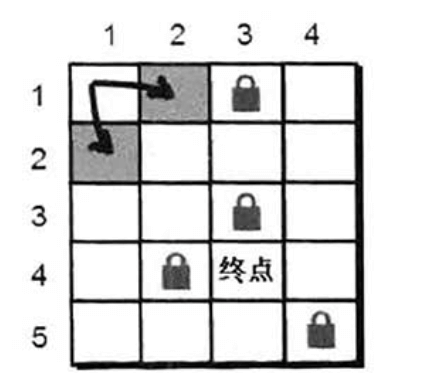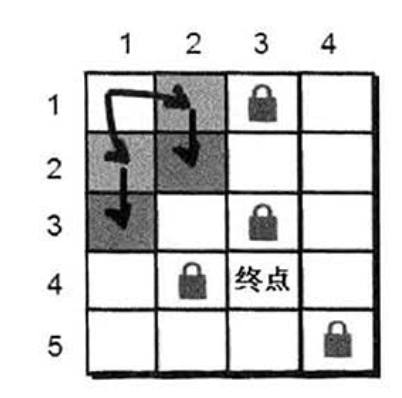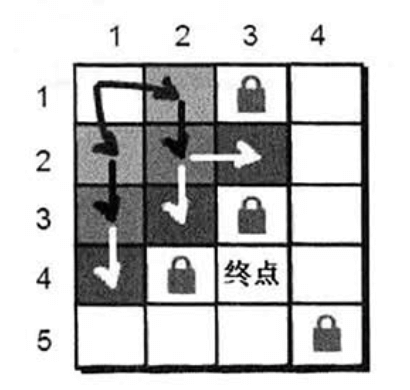1
2
3
4
5
6
7
8
9
10
11
12
13
14
15
16
17
18
19
20
21
22
23
24
25
26
27
28
29
30
31
32
33
34
35
36
37
38
39
40
41
42
43
44
45
46
47
48
49
50
51
52
53
54
55
56
57
58
59
60
61
62
63
64
65
66
67
68
69
70
71
72
73
74
75
76
77
78
79
80
81
82
83
84
#include <stdio.h>
struct note
{
int x;
int y;
int f;//父亲在队列中的编号
int s;//步数
};
int main()
{
struct note que;//假设地图大小不超过50*50
int a={0},book={0};
//定义一个用于表示走的方向的数组
int next = {
{0,1},
{1,0},
{0,-1},
{-1,0}
};
int i,j,k,n,m,startx,starty,p,q,tx,ty,flag;

scanf("%d %d",&n,&m);
for(i=1;i<=n;i++)
for(j=1;j<=m;j++)
scanf("%d",&a[i][j]);
scanf("%d %d %d %d",&startx,&starty,&p,&q);

//队列初始化
tail=1;
//往队列插入迷宫入口坐标
que[tail].x=startx;
que[tail].y=starty;
que[tail].f=0;
que[tail].s=0;
tail++;
book[startx][starty]=1;

flag=0;//用来标记是否到达目标点，0表示暂时还没有到达，1表示到达
//当队列不为空的时候循环
{
//枚举4个方向
for(k=0;k<=3;k++)
{
//计算下一个点的坐标
//判断是否越界
if(tx<1 || tx>n || ty<1 || ty>m)
continue;;
//判断是否是障碍物或者已经在路径中
if(a[tx][ty]==0 && book[tx][ty]==0)
{
//把这个点标记为已经走过
//注意宽搜每个点只入队一次，所以和深搜不同，不需要将book数组还原
book[tx][ty]=1;
//插入新的点到队列中
que[tail].x=tx;
que[tail].y=ty;
tail++;
}
//如果到目标点了，停止扩展，任务结束，退出循环
if(tx==p && ty==q)
{
flag=1;
break;
}
}
if(flag==1)
break;
}

//打印队列中末尾最后一个点（目标点）的步数
//注意tail是指向队列队尾（即最后一位）的下一个位置，所以这需要-1
printf("%d",que[tail-1].s);

getchar();getchar();
return 0;
}


1
2
3
4
5
6
7
5 4
0 0 1 0
0 0 0 0
0 0 1 0
0 1 0 0
0 0 0 1
1 1 4 3


1
7


# 4.再解炸弹人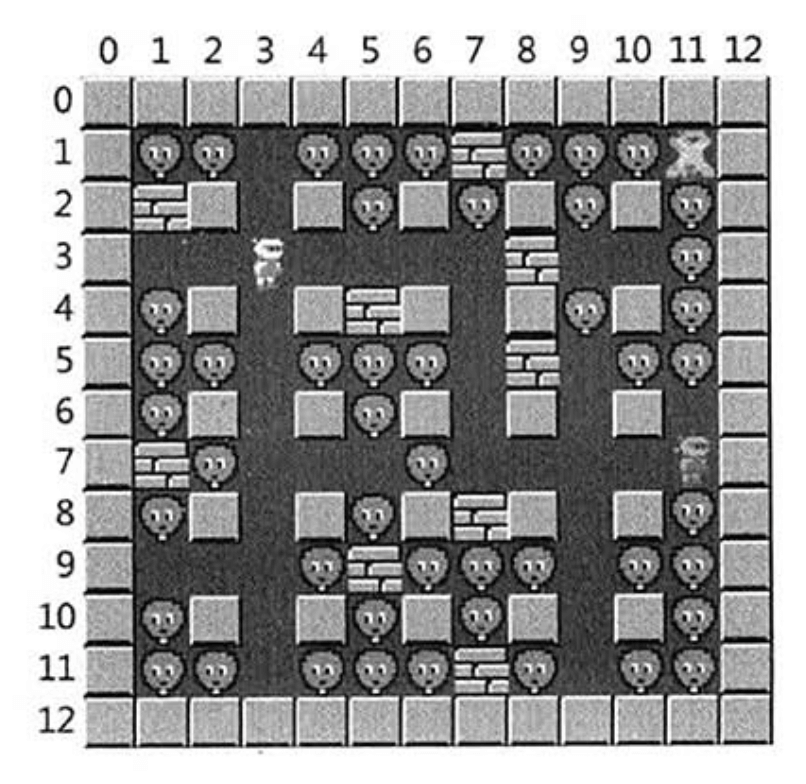1
2
3
4
5
6
7
8
9
10
11
12
13
14
15
16
17
18
19
20
21
22
23
24
25
26
27
28
29
30
31
32
33
34
35
36
37
38
39
40
41
42
43
44
45
46
47
48
49
50
51
52
53
54
55
56
57
58
59
60
61
62
63
64
65
66
67
68
69
70
71
72
73
74
75
76
77
78
79
80
81
82
83
84
85
86
87
88
89
90
91
92
93
94
95
96
97
98
99
100
101
102
103
104
105
106
107
108
109
110
111
112
113
114
115
116
117
118
119
120
121
122
123
124
125
126
#include <stdio.h>
struct note
{
int x;
int y;
};
char a;//用来存储地图
int getnum(int i, int j)
{
int sum,x,y;
sum=0;//sum用来计数（可以消灭的敌人数），所以需要初始化为0
//将坐标i,j复制到两个新变量x,y中，以便之后向上下左右四个方向统计可以消灭的敌人数

x=i;y=j;
while(a[x][y]!='#')//判断是不是墙，如果不是墙就继续
{
//如果当前的点是敌人，则进行计数
if(a[x][y]=='G')
sum++;
x--;
}

x=i;y=j;
while(a[x][y]!='#')//判断是不是墙，如果不是墙就继续
{
//如果当前的点是敌人，则进行计数
if(a[x][y]=='G')
sum++;
x++;
}

x=i;y=j;
while(a[x][y]!='#')//判断是不是墙，如果不是墙就继续
{
//如果当前的点是敌人，则进行计数
if(a[x][y]=='G')
sum++;
y--;
}

x=i;y=j;
while(a[x][y]!='#')//判断是不是墙，如果不是墙就继续
{
//如果当前的点是敌人，则进行计数
if(a[x][y]=='G')
sum++;
y++;
}

return sum;
}

int main()
{
struct note que;//假设地图大小不超过20*20
int book={0};//定义一个标记数组并全部初始化为0
int i,j,k,sum,max=0,mx,my,n,m,startx,starty,tx,ty;

//定义一个用于表示走的方向的数组
int next = {
{0,1},
{1,0},
{0,-1},
{-1,0}
};

//n行m列
scanf("%d %d %d %d",&n,&m,&startx,&starty);

//读入n行字符
for(i=0;i<=n-1;i++)
scanf("%s",a[i]);

//队列初始化
tail=1;
//往队列插入小人的起始坐标
que[tail].x=startx;
que[tail].y=starty;
tail++;
book[startx][starty]=1;
max=getnum(startx, starty);
mx=startx;
my=starty;
//当队列不为空的时候循环
{
//枚举4个方向
for(k=0;k<=3;k++)
{
//尝试走的下一个点的坐标

//判断是否越界
if(tx<0 || tx>n-1 || ty<0 || ty>m-1)
continue;

//判断是否为平地或者曾经走过
if(a[tx][ty]=='.' && book[tx][ty]==0)
{
//每个点只入队一次，所以需要标记这个点已经走过
book[tx][ty]=1;
//插入新扩展的点到队列中
que[tail].x=tx;
que[tail].y=ty;
tail++;

//统计当前新扩展的点可以消灭的敌人总数
sum=getnum(tx, ty);
//更新max的值
if(sum>max)
{
max=sum;
mx=tx;
my=ty;
}
}
}
}
printf("将炸弹放置在(%d,%d)处，可以消灭%d个敌人\n",mx,my,max);
getchar();getchar();
return 0;
}


1
2
3
4
5
6
7
8
9
10
11
12
13
14
13 13 3 3
#############
#GG.GGG#GGG.#
###.#G#G#G#G#
#.......#..G#
#G#.###.#G#G#
#GG.GGG.#.GG#
#G#.#G#.#.#.#
##G...G.....#
#G#.#G###.#G#
#...G#GGG.GG#
#G#.#G#G#.#G#
#GG.GGG#G.GG#
#############


1



1
2
3
4
5
6
7
8
9
10
11
12
13
14
15
16
17
18
19
20
21
22
23
24
25
26
27
28
29
30
31
32
33
34
35
36
37
38
39
40
41
42
43
44
45
46
47
48
49
50
51
52
53
54
55
56
57
58
59
60
61
62
63
64
65
66
67
68
69
70
71
72
73
74
75
76
77
78
79
80
81
82
83
84
85
86
87
88
89
90
91
92
93
94
95
96
97
98
99
100
101
102
103
104
105
106
107
108
#include <stdio.h>
char a;//用来存储地图
int book,max,mx,my,n,m;
int getnum(int i, int j)
{
int sum,x,y;
sum=0;//sum用来计数（可以消灭的敌人数），所以需要初始化为0
//将坐标i,j复制到两个新变量x,y中，以便之后向上下左右四个方向统计可以消灭的敌人数

x=i;y=j;
while(a[x][y]!='#')//判断是不是墙，如果不是墙就继续
{
//如果当前的点是敌人，则进行计数
if(a[x][y]=='G')
sum++;
x--;
}

x=i;y=j;
while(a[x][y]!='#')//判断是不是墙，如果不是墙就继续
{
//如果当前的点是敌人，则进行计数
if(a[x][y]=='G')
sum++;
x++;
}

x=i;y=j;
while(a[x][y]!='#')//判断是不是墙，如果不是墙就继续
{
//如果当前的点是敌人，则进行计数
if(a[x][y]=='G')
sum++;
y--;
}

x=i;y=j;
while(a[x][y]!='#')//判断是不是墙，如果不是墙就继续
{
//如果当前的点是敌人，则进行计数
if(a[x][y]=='G')
sum++;
y++;
}

return sum;
}

void dfs(int x,int y)
{
//定义一个用于表示走的方向的数组
int next = {
{0,1},
{1,0},
{0,-1},
{-1,0}
};
int k,sum,tx,ty;

//计算当前这个点可以消灭的敌人总数
sum=getnum(x, y);
//更新max的值
if(sum>max)
{
max=sum;
mx=x;
my=y;
}

//枚举4个方向
for(k=0;k<=3;k++)
{
tx=x+next[k];
ty=y+next[k];
//判断是否越界
if(tx<0 || tx>n-1 || ty<0 || ty>m-1)
continue;
if(a[tx][ty]=='.' && book[tx][ty]==0)
{
book[tx][ty]=1;//标记这个点已走过
dfs(tx,ty);//开始尝试下一个点
}
}
return;
}

int main()
{
int i,startx,starty;

//n行m列
scanf("%d %d %d %d",&n,&m,&startx,&starty);

//读入n行字符
for(i=0;i<=n-1;i++)
scanf("%s",a[i]);

//从小人所站的位置开始尝试
book[startx][starty]=1;
max=getnum(startx, starty);
mx=startx;
my=starty;
dfs(startx, starty);

printf("将炸弹放置在(%d,%d)处，可以消灭%d个敌人\n",mx,my,max);
getchar();getchar();
return 0;
}


# 5.宝岛探险

1
2
3
4
5
6
7
8
9
10
11
12
13
14
15
16
17
18
19
20
21
22
23
24
25
26
27
28
29
30
31
32
33
34
35
36
37
38
39
40
41
42
43
44
45
46
47
48
49
50
51
52
53
54
55
56
57
58
59
60
61
62
63
64
65
66
67
68
69
70
71
72
73
74
#include <stdio.h>
struct note
{
int x;
int y;
};
int main()
{
struct note que;//假设地图大小不超过50*50
int a;
int book={0};
int i,j,k,sum,max=0,mx,my,n,m,startx,starty,tx,ty;

//定义一个方向数组
int next = {
{0,1},
{1,0},
{0,-1},
{-1,0}
};

//读入n行m列以及小哼降落的坐标
scanf("%d %d %d %d",&n,&m,&startx,&starty);

//读入地图
for(i=1;i<=n;i++)
for(j=1;j<=n;j++)
scanf("%d",&a[i][j]);

//队列初始化
tail=1;
//往队列插入降落的起始坐标
que[tail].x=startx;
que[tail].y=starty;
tail++;
book[startx][starty]=1;
sum=1;

//当队列不为空的时候循环
{
//枚举4个方向
for(k=0;k<=3;k++)
{
//计算下一步的坐标

//判断是否越界
if(tx<1 || tx>n || ty<1 || ty>m)
continue;

//判断是否是陆地或者曾经是否走过
if(a[tx][ty]>0 && book[tx][ty]==0)
{
sum++;
//每个点只入队一次，所以需要标记这个点已经走过
book[tx][ty]=1;
//将新扩展的点加入队列
que[tail].x=tx;
que[tail].y=ty;
tail++;
}
}
}
//最后输出岛屿的大小
printf("%d\n",sum);

getchar();getchar();
return 0;
}


1
2
3
4
5
6
7
8
9
10
11
10 10 6 8
1 2 1 0 0 0 0 0 2 3
3 0 2 0 1 2 1 0 1 2
4 0 1 0 1 2 3 2 0 1
3 2 0 0 0 1 2 4 0 0
0 0 0 0 0 0 1 5 3 0
0 1 2 1 0 1 5 4 3 0
0 1 2 3 1 3 6 2 1 0
0 0 3 4 8 9 7 5 0 0
0 0 0 3 7 8 6 0 1 2
0 0 0 0 0 0 0 0 1 0


1
38


1
2
3
4
5
6
7
8
9
10
11
12
13
14
15
16
17
18
19
20
21
22
23
24
25
26
27
28
29
30
31
32
33
34
35
36
37
38
39
40
41
42
43
44
45
46
47
48
49
50
51
#include <stdio.h>
int a;
int book,n,m,sum;
void dfs(int x, int y)
{
//定义一个方向数组
int next = {
{0,1},
{1,0},
{0,-1},
{-1,0}
};
int k,tx,ty;

//枚举4个方向
for(k=0;k<=3;k++)
{
//计算下一步的坐标
tx=x+next[k];
ty=y+next[k];
//判断是否越界
if(tx<1 || tx>n || ty<1 || ty>m)
continue;
//判断是否是陆地
if(a[tx][ty]>0 && book[tx][ty]==0)
{
sum++;
book[tx][ty]=1;//标记这个点已走过
dfs(tx,ty);//开始尝试下一个点
}
}
return;
}

int main()
{
int i,j,startx,starty;
scanf("%d %d %d %d",&n,&m,&startx,&starty);
//读入地图
for(i=1;i<=n;i++)
for(j=1;j<=n;j++)
scanf("%d",&a[i][j]);
book[startx][starty]=1;
sum=1;
//从降落的位置开始
dfs(startx, starty);
//最后输出岛屿的大小
printf("%d\n",sum);
getchar();getchar();
return 0;
}


1
2
3
4
5
6
7
8
9
10
11
12
13
14
15
16
17
18
19
20
21
22
23
24
25
26
27
28
29
30
31
32
33
34
35
36
37
38
39
40
41
42
43
44
45
46
47
48
49
50
51
52
53
54
55
56
57
58
59
60
61
#include <stdio.h>
int a;
int book,n,m,sum;
void dfs(int x, int y, int color)
{
//定义一个方向数组
int next = {
{0,1},
{1,0},
{0,-1},
{-1,0}
};
int k,tx,ty;
a[x][y]=color;//对a[x][y]这个格子进行染色

//枚举4个方向
for(k=0;k<=3;k++)
{
//计算下一步的坐标
tx=x+next[k];
ty=y+next[k];
//判断是否越界
if(tx<1 || tx>n || ty<1 || ty>m)
continue;
//判断是否是陆地
if(a[tx][ty]>0 && book[tx][ty]==0)
{
sum++;
book[tx][ty]=1;//标记这个点已走过
dfs(tx,ty,color);//开始尝试下一个点
}
}
return;
}

int main()
{
int i,j,startx,starty;
scanf("%d %d %d %d",&n,&m,&startx,&starty);
//读入地图
for(i=1;i<=n;i++)
for(j=1;j<=n;j++)
scanf("%d",&a[i][j]);
book[startx][starty]=1;
sum=1;
//从降落的位置开始
dfs(startx, starty,-1);

//输出已经染色后的地图
for(i=1;i<=n;i++)
{
for(j=1;j<=m;j++)
{
printf("%3d",a[i][j]);
}
printf("\n");
}

getchar();getchar();
return 0;
}


1
2
3
4
5
6
7
8
9
10
11
10 10 6 8
1 2 1 0 0 0 0 0 2 3
3 0 2 0 1 2 1 0 1 2
4 0 1 0 1 2 3 2 0 1
3 2 0 0 0 1 2 4 0 0
0 0 0 0 0 0 1 5 3 0
0 1 2 1 0 1 5 4 3 0
0 1 2 3 1 3 6 2 1 0
0 0 3 4 8 9 7 5 0 0
0 0 0 3 7 8 6 0 1 2
0 0 0 0 0 0 0 0 1 0


1
2
3
4
5
6
7
8
9
10
1  2  1  0  0  0  0  0  2  3
3  0  2  0 -1 -1 -1  0  1  2
4  0  1  0 -1 -1 -1 -1  0  1
3  2  0  0  0 -1 -1 -1  0  0
0  0  0  0  0  0 -1 -1 -1  0
0 -1 -1 -1  0 -1 -1 -1 -1  0
0 -1 -1 -1 -1 -1 -1 -1 -1  0
0  0 -1 -1 -1 -1 -1 -1  0  0
0  0  0 -1 -1 -1 -1  0  1  2
0  0  0  0  0  0  0  0  1  0


1
2
3
4
5
6
7
8
9
10
11
12
13
14
15
16
17
18
19
20
21
22
23
24
25
26
27
28
29
30
31
32
33
34
35
36
37
38
39
40
41
42
43
44
45
46
47
48
49
50
51
52
53
54
55
56
57
58
59
60
61
62
63
64
65
66
67
68
69
70
71
72
73
74
#include <stdio.h>
int a;
int book,n,m,sum;
void dfs(int x, int y, int color)
{
//定义一个方向数组
int next = {
{0,1},
{1,0},
{0,-1},
{-1,0}
};
int k,tx,ty;
a[x][y]=color;//对a[x][y]这个格子进行染色

//枚举4个方向
for(k=0;k<=3;k++)
{
//计算下一步的坐标
tx=x+next[k];
ty=y+next[k];
//判断是否越界
if(tx<1 || tx>n || ty<1 || ty>m)
continue;
//判断是否是陆地
if(a[tx][ty]>0 && book[tx][ty]==0)
{
sum++;
book[tx][ty]=1;//标记这个点已走过
dfs(tx,ty,color);//开始尝试下一个点
}
}
return;
}

int main()
{
int i,j,num=0;
scanf("%d %d",&n,&m);
//读入地图
for(i=1;i<=n;i++)
for(j=1;j<=n;j++)
scanf("%d",&a[i][j]);

//对每一个大于0的点尝试进行dfs染色
for(i=1;i<=n;i++)
{
for(j=1;j<=m;j++)
{
if(a[i][j]>0)
{
num--;//小岛需要染的颜色的编号
//每发现一个小岛应染以不同的颜色，因此每次要-1
book[i][j]=1;
dfs(i, j, num);
}
}
}

//输出已经染色后的地图
for(i=1;i<=n;i++)
{
for(j=1;j<=m;j++)
{
printf("%3d",a[i][j]);
}
printf("\n");
}
//输出小岛的个数
printf("有%d个小岛\n",-num);

getchar();getchar();
return 0;
}


1
2
3
4
5
6
7
8
9
10
11
10 10
1 2 1 0 0 0 0 0 2 3
3 0 2 0 1 2 1 0 1 2
4 0 1 0 1 2 3 2 0 1
3 2 0 0 0 1 2 4 0 0
0 0 0 0 0 0 1 5 3 0
0 1 2 1 0 1 5 4 3 0
0 1 2 3 1 3 6 2 1 0
0 0 3 4 8 9 7 5 0 0
0 0 0 3 7 8 6 0 1 2
0 0 0 0 0 0 0 0 1 0


1
2
3
4
5
6
7
8
9
10
11
-1 -1 -1  0  0  0  0  0 -2 -2
-1  0 -1  0 -3 -3 -3  0 -2 -2
-1  0 -1  0 -3 -3 -3 -3  0 -2
-1 -1  0  0  0 -3 -3 -3  0  0
0  0  0  0  0  0 -3 -3 -3  0
0 -3 -3 -3  0 -3 -3 -3 -3  0
0 -3 -3 -3 -3 -3 -3 -3 -3  0
0  0 -3 -3 -3 -3 -3 -3  0  0
0  0  0 -3 -3 -3 -3  0 -4 -4
0  0  0  0  0  0  0  0 -4  0



# 6.水管工游戏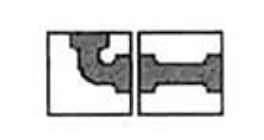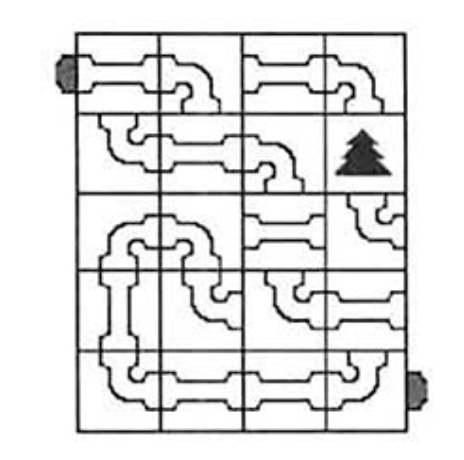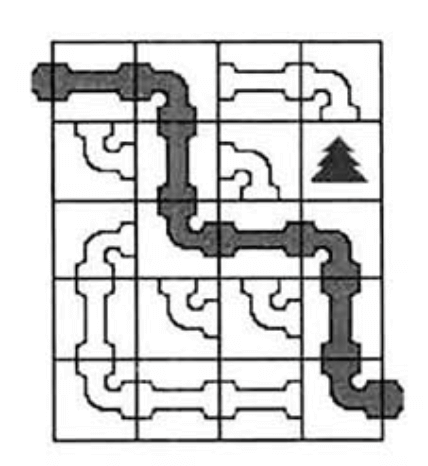1
2
3
4
5
6
5 4
5 3 5 3
1 5 3 0
2 3 5 1
6 1 1 5
1 5 5 4


1
(1,1) (1,2) (2,2) (3,2) (3,3) (3,4) (4,4) (5,4)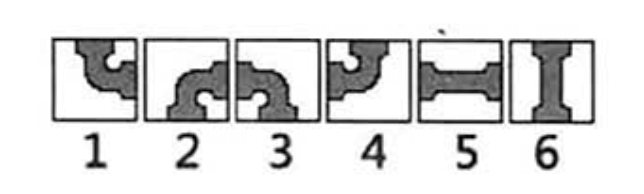1
2
3
4
5
6
7
8
9
10
11
12
13
14
15
16
17
18
19
20
21
22
23
24
25
26
27
28
29
30
31
32
33
34
35
36
37
38
39
40
41
42
43
44
45
46
47
48
49
50
51
52
53
54
55
56
57
58
59
60
61
62
63
64
65
66
67
68
69
70
71
72
73
74
75
76
77
78
79
80
81
82
83
84
85
86
87
88
89
90
91
92
93
#include <stdio.h>
int a;//假设土地的大小不超过50*50
int book,n,m,flag=0;
struct note
{
int x;
int y;
} s;
int top=0;
void dfs(int x, int y, int front)
{
int i;
//判断是否到达终点
if(x==n && y==m+1)
{
flag=1;//找到铺设方案
for(i=1;i<=top;i++)
printf("(%d,%d) ",s[i].x,s[i].y);
return;
}
//判断是否越界
if(x<1 || x>n || y<1 || y>m)
return;
//判断这个管道是否在路径中已经使用过
if(book[x][y]==1)
return;
book[x][y]=1;//标记使用当前这个管道

//将当前尝试的坐标入栈
top++;
s[top].x=x;
s[top].y=y;

//当前水管是直管的情况
if(a[x][y]>=5 && a[x][y]<=6)
{
if(front==1)//进水口在左边的情况
dfs(x,y+1,1);//只能使用5号这种摆放方式
if(front==2)//进水口在上边的情况
dfs(x+1,y,2);//只能使用6号这种摆放方式
if(front==3)//进水口在右边的情况
dfs(x,y-1,3);//只能使用5号这种摆放方式
if(front==4)//进水口在下边的情况
dfs(x-1,y,4);//只能使用6号这种摆放方式
}

//当前水管是弯管的情况
if(a[x][y]>=1 && a[x][y]<=4)
{
if(front==1)
{
dfs(x+1,y,2);//3号状态
dfs(x-1,y,4);//4号状态
}
if(front==2)
{
dfs(x,y+1,1);//1号状态
dfs(x,y-1,3);//4号状态
}
if(front==3)
{
dfs(x-1,y,4);//1号状态
dfs(x+1,y,2);//2号状态
}
if(front==4)
{
dfs(x,y+1,1);//2号状态
dfs(x,y-1,3);//3号状态
}
}

book[x][y]=0;//取消标记
top--;//将当前尝试的坐标出栈
return;
}

int main()
{
int i,j,num=0;
scanf("%d %d",&n,&m);
//读入游戏地图
for(i=1;i<=n;i++)
for(j=1;j<=m;j++)
scanf("%d",&a[i][j]);
//开始搜索，从1,1点开始，进水方向也是1
dfs(1,1,1);
//判断是否找到铺设方案
if(flag==0)
printf("impossible\n");

getchar();getchar();
return 0;
}